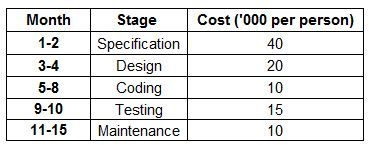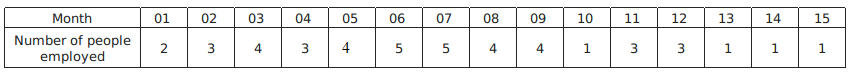### CAT 1996 Question Paper Question 56

Instructions

Answer the questions based on the following information. Mulayam Software Co., before selling a package to its clients, follows the given schedule.The number of people employed in each month is:Question 56

# Due to overrun in ‘design’, the design stage took 3 months, i.e. months 3, 4 and 5. The number of people working on design in the fifth month was 5. Calculate the percentage change in the cost incurred in the fifth month. (Due to improvement in ‘coding’ technique, this stage was completed in months 6-8 only.)

Solution

In given table, value of cost for 5th month = $$(N) \times (C)$$ (No.of employer for 5th month = N and cost per person for 5th month = C)
So value of cost for 5th month before overrun = $$4 \times 10 = 40$$
value of cost for 5th month after overrun = $$5 \times 20 = 100$$
Change = 60
%change = $$\frac{60}{40} \times 100$$ = 150

• All Quant CAT Formulas and shortcuts PDF
• 30+ CAT previous papers with solutions PDF